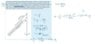# Centripetal Acceleration

• Jpyhsics
In summary, a centrifuge is a laboratory device used for spinning samples of material. The equation T=2πr/f cannot be correct as you can tell by doing a unit analysis of the equation.

## Homework Statement

A centrifuge is a laboratory device used for spinning samples of material. In a blood centrifuge, a test tube is inserted at an angle θ=32.0° with respect to the vertical and the whole sample is spun at high speed. For a typical test that is l= 15.9 cm long and is spun in a centrifuge at a rate of 4316 rotations per minute, what is the centripetal acceleration experienced by the sample at a point x=9.93 cm from the rotation axis, as measured along the test tube? Express your answer in units of the standard gravity g. Thus, if your answer is 19.62 m/s2 = 2.00×9.81 m/s2, you would enter 2.00 into the box.

a=v2/r
T=2πr/f
v=d/t

## The Attempt at a Solution

First, I found the period
T=2π(15.9)/(4316/60)=1.3888
v=2π(9.93)/1.3888=71.9
a=(71.9)2/9.93

I don't think I did this correctly, as I didnt use the angle...
I have included a photo as well,

#### Attachments

•IMG_D85CAED94C46-1.jpeg
25.2 KB · Views: 280
Jpyhsics said:

## Homework Equations

a=v2/r
T=2πr/f
v=d/t
The equation T=2πr/f cannot be correct as you can tell by doing a unit analysis of the equation.

3. The Attempt at a Solution
First, I found the period
T=2π(15.9)/(4316/60)=1.3888
v=2π(9.93)/1.3888=71.9
a=(71.9)2/9.93
You need to be clear on the meaning of r in the equations. You want the centripetal acceleration of a particular point in the test tube. Mark this point in your diagram and try to draw a representation of r in the diagram.

TSny said:
The equation T=2πr/f cannot be correct as you can tell by doing a unit analysis of the equation.

You need to be clear on the meaning of r in the equations. You want the centripetal acceleration of a particular point in the test tube. Mark this point in your diagram and try to draw a representation of r in the diagram.
So how what formulas would I have to use?
I would assume ac=v2/r? but what about the period (T)?

Jpyhsics said:
So how what formulas would I have to use?
Would I use the 15.9cm?

Jpyhsics said:
So how what formulas would I have to use?
You should be able to solve it using your two equations

a=v2/r
v=d/t

But if you have learned how to write centripetal acceleration in terms of angular velocity ω, you might find that to be a quicker way to the answer.

Jpyhsics said:
but what about the period (T)?
If the test tube makes 4316 revolutions in one minute, how much time does it take for it to make one revolution?

TSny said:
If the test tube makes 4316 revolutions in one minute, how much time does it take for it to make one revolution?
0.0139s per rotation?

Jpyhsics said:
0.0139s per rotation?
Oh I see how the angle relates now. I got an answer of 10749 m/s2. Thank you so much for your help! :)

Jpyhsics said:
Oh I see how the angle relates now. I got an answer of 10749 m/s2. Thank you so much for your help! :)